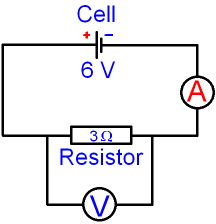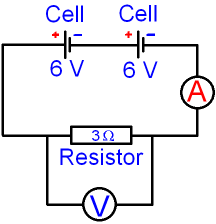gcsescience.com                                       19                                       gcsescience.com

Electricity

Series Circuits

What happens to the Voltage and Current
in a Circuit when Cells are connected in Series?

The current in the circuit below can be calculated
using the equation
voltage = current x resistance   V = I x R

The equation can be rearranged to give
current = voltage ÷ r
esistance    I = V ÷ RIn the circuit above, V = 6 volts and R = 3 ohms.

I = V ÷ R
= 6 ÷ 3
= 2 amps.

What happens to the Voltage and Current
in a Circuit if Two Cells are connected in Series?

If an identical cell is placed in series with
the original cell, the current in the circuit doubles
because the total voltage of the circuit doubles.In the circuit above,
R = 3 ohms and V = 6 + 6 volts = 12 volts.

I = V ÷ R
= 12 ÷ 3
= 4 amps.

Cells connected together are called a battery.
If more cells (or batteries) are connected together
in series then the total supply voltage is the
sum of the individual voltages for each cell if they are
connected the right way round, plus to minus.

Compare this with cells in parallel.

gcsescience.com         Physics Quiz         Index         Electricity Quiz         gcsescience.com